Technical Article

# The Basics of SSRs (Solid-State Relays): The Switching Device

June 16, 2017 by Dr. Steve Arar

## An introduction to the basic operation of solid-state relays with a focus on the output devices in today’s SSRs.

This article provides an introduction to the basic operation of solid-state relays with a focus on the output devices in today’s SSRs.

There are many circumstances in which we need to control a high current/voltage load based on the operation of a low-power circuit, such as when using the 5V output of a microcontroller to turn on a 10A, 240V load. In these cases, it is necessary to provide sufficient isolation between the high-power and low-power parts of the system. Different types of relays such as electromechanical relays (EMR), reed relays, and solid-state relays (SSR) can be used to achieve this goal.

While EMRs are still widely used, they have several disadvantages in comparison to SSRs. This article briefly reviews the drawbacks of EMRs and gives some details regarding the basic operation of SSRs, with emphasis on the output devices.

### EMRs and Their Drawbacks

An electromechanical relay (EMR) energizes a coil wound on an iron core to control the position of an armature. For a normally open output, the energized coil forces the armature to put the electrical contacts into the ON state. When the coil is de-energized, springs can move the contacts back to the OFF position.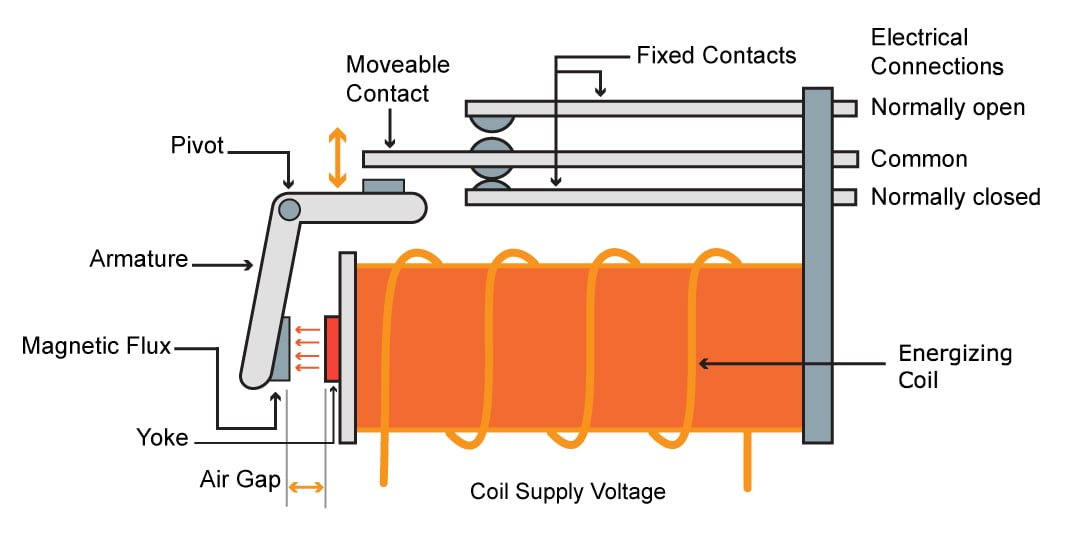##### Figure 1. In an EMR, the energized coil moves the armature to either connect or disconnect the output terminals.

An electromechanical relay is robust and versatile. However, it takes up more room and is slower than an SSR. Typically, an EMR needs 5 to 15 ms to switch and settle—a delay which is not acceptable in some applications. Moreover, due to their moving parts, EMRs have a shorter operational lifetime.

An electromechanical relay uses magnetic fields to provide isolation; an SSR, in contrast, achieves this goal generally through opto-coupling. As shown in Figure 2, in an SSR a small input voltage, typically 3 to 32 VDC, is used to illuminate an LED. When the LED is turned on, an output photo-sensitive device, such as a TRIAC, turns on and conducts current.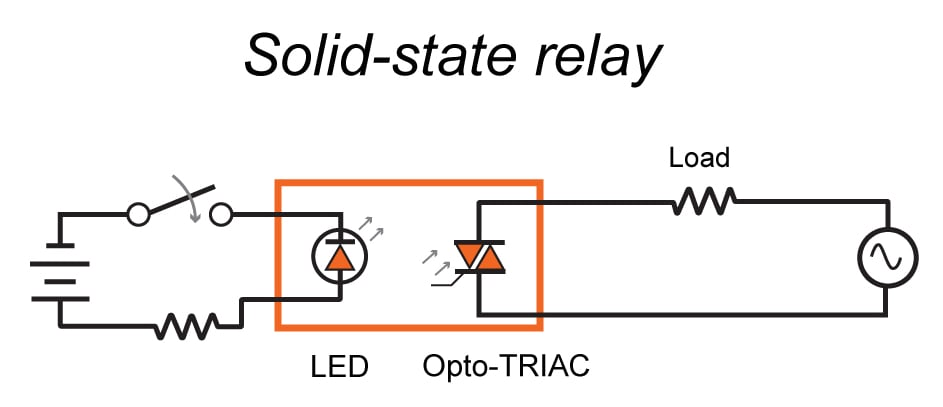##### Figure 2. The basic structure of an SSR. Image adapted from pc-control.

An SSR can be designed to switch a DC or an AC load, and some types are capable of switching both AC and DC loads. An SSR's output type (AC, DC, or AC/DC) is determined by the type of switching device: a transistor (either bipolar or MOS), an SCR, or a TRIAC.

### BJT-Based SSRs

The simplified output structure of these SSRs is shown in Figure 3 (please note that this is only the output device and the opto-coupler is not shown). When the LED is on, the transistor starts conducting the current. As shown in Figure 3, the SSR has load flexibility: RL can be connected to either the collector (RL in Figures 3 and 5) or the emitter (RL (ALT) in Figures 3 and 5) terminal of the transistor. Considering the well-known I-V characteristic curves of a BJT, shown in Figure 4, it is desired to have the transistor operating in A (saturation) or B (almost completely off).

As the transistor's operating point moves farther away from A or B and toward the center of the load line, the transistor enters a region in which both the collector-emitter voltage ($$V_{CE}$$) and the collector current ($$I_{C}$$) are high. This results in high power dissipation that may damage the SSR (at A and B, either $$V_{CE}$$ or $$I_C$$ is small and the produced heat can be handled). To hasten the transition between ON and OFF states, some SSRs use positive feedback.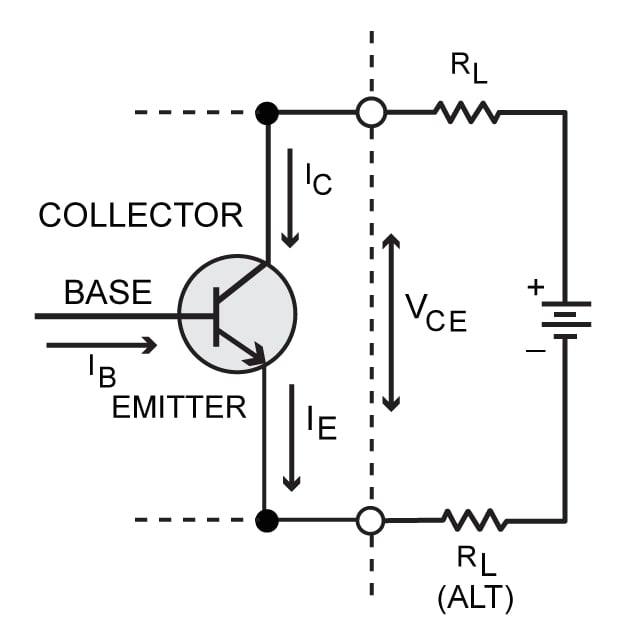##### Figure 3. Using a BJT as the output device of an SSR. Image adapted from Solid-state Relay Handbook with Applications by Anthony Bishop.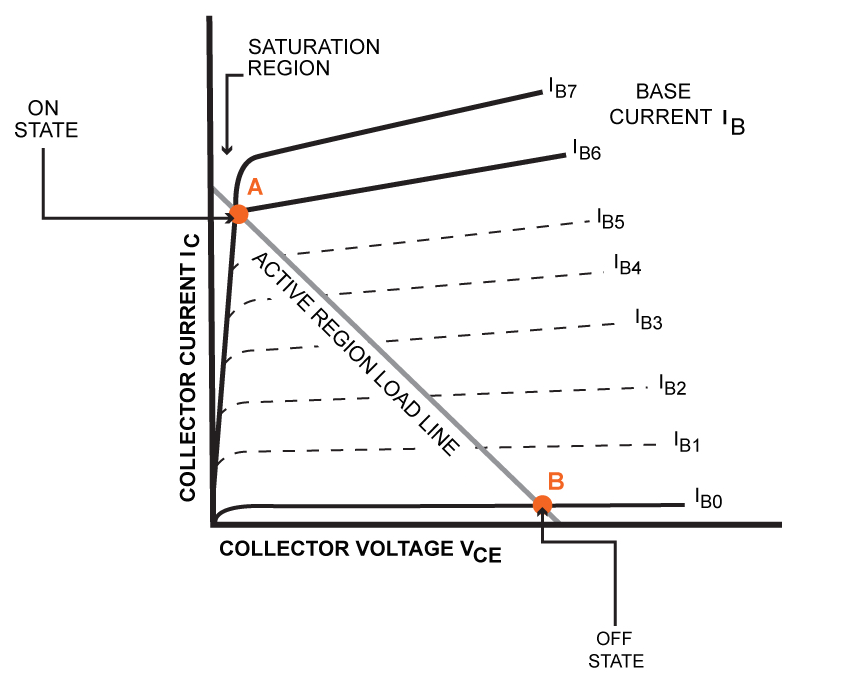##### Figure 4. Current-voltage characteristics of a BJT. Image adapted from Solid-state Relay Handbook with Applications by Anthony Bishop.

When the switching device of an SSR is on (see point A above), there is a voltage drop across the SSR output terminals which is called the “on-state voltage drop”. This parameter is given in the device datasheet. For BJT-based SSRs, such as the DC60 series, the maximum on-state voltage drop might be 1 to 1.3 V. Assuming a 1 V drop at the SSR output, we can estimate the power dissipation of a BJT-based SSR to be about 1 watt per ampere.

With BJT SSRs, to achieve a higher gain from photo-coupler to the switching stage, manufacturers employ structures such as the Darlington and complementary configurations, as shown below.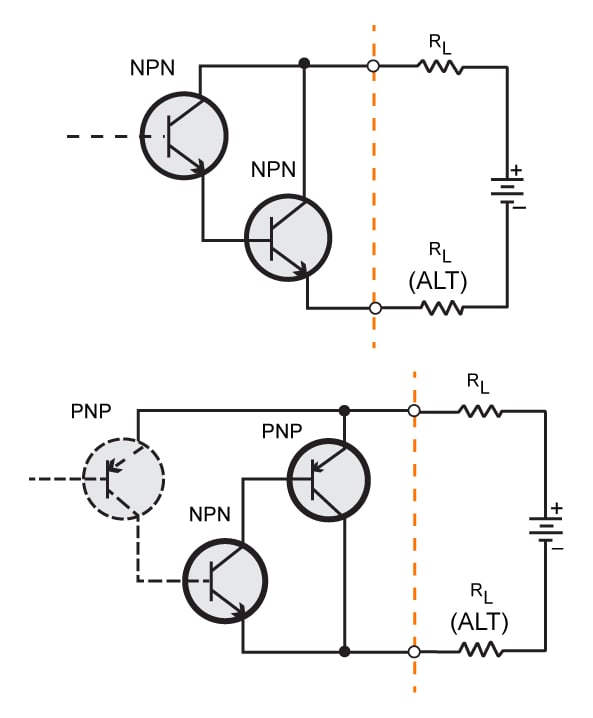### MOS-Based SSRs

Many SSRs use MOS transistors as the switching device. Figure 6 shows an example of SSRs that can be used for both AC and DC loads. Why do these SSRs need two transistors? Using only a single transistor, the SSR cannot block the AC voltage properly. This is due to the fact that, as shown in Figure 6, each MOS transistor generally has a parasitic diode, called a body diode, between the drain and source.

With only one transistor between terminals 4 and 3 in Figure 6, the body diode will conduct for half the cycle with an AC supply. Therefore, while the current cannot flow through the FET itself, the diode is on for half the cycle and allows current to pass through the device. To circumvent this problem, two transistors are used in series so that in each half cycle there is one reverse-biased diode and the current is blocked.

What is the origin of this diode? Each MOS transistor has four terminals: source, drain, gate, and body. For an NMOS, the source and drain are N type and the body is P doped. Hence, we have one diode between source and body and another one between drain and body. Since the source is generally connected to the body (especially in power MOSFETs), there remains a single diode between the body (which is connected to the source) and the drain. During normal operation of the N-channel transistor where the drain is at a higher voltage than the source, the diode is reverse biased. However, if the source is connected to a higher voltage than the drain terminal, the diode will start conducting current.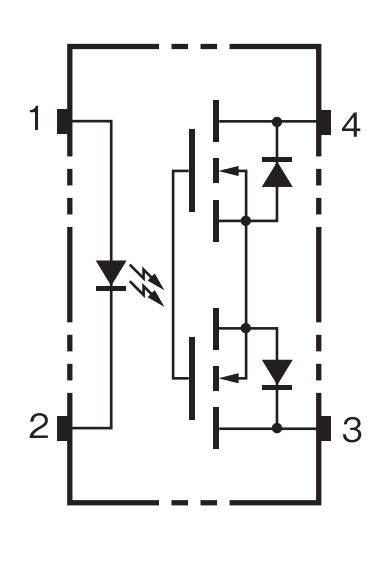##### Figure 6. An MOS-based SSR. Image courtesy of Omron.

While datasheets for BJT-based SSRs specify the on-state voltage drop, datasheets for MOS-based SSRs give the on-state resistance, which varies significantly from one device to another and can be as low as a few milliohms.

Some MOS-based SSRs provide a lead to the source terminal. This allows for two different configurations, as shown in Figure 7. The DC-only configuration of Figure 7 can have almost half the resistance of the AC/DC configuration.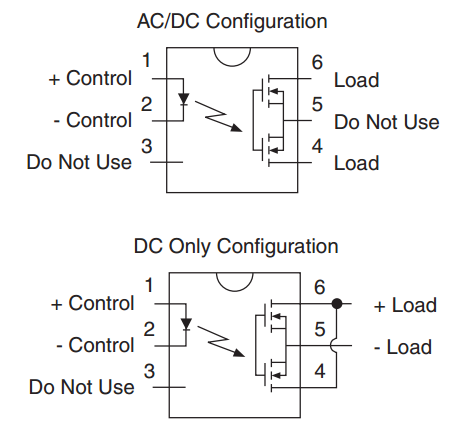##### Figure 7. AC/DC and DC-only configuration of a MOS-based SSR. Image courtesy of IXYS (PDF).

High-voltage/current AC SSRs usually utilize SCRs or TRIACs as the switching device. These AC SSRs can be used to switch a 90 A, 480 Vrms load with only a 50 mA gate drive. Unlike the previously discussed switching devices, SCRs and TRIACs cannot be used for DC switching­­. This is due to a positive feedback mechanism which is present in SCRs and TRIACs and prevents the device from turning off unless the current passing through the device goes below a certain level (and this would not typically occur with a DC load). The main characteristics of these SSRs are briefly discussed in the rest of the article.

### SCR- and TRIAC-Based SSRs

A silicon controlled rectifier (SCR) is a four-layer PNPN structure with three terminals: anode, gate, and cathode. An SCR incorporates regenerative feedback such that it has two stable states: ON and OFF. When an SCR is off, it blocks current in both directions, and when turned on, the SCR acts as a rectifier and allows the current to flow from anode to cathode. The operation of an SCR can be understood by comparing the PNPN structure of an SCR with the transistor analogy shown in Figure 8. Note that the transistor analogy in Figure 8(b) has two three-layer elements (a PNP transistor and an NPN one), but considering the connection between layers, shown in Figure 8(a), we can share some of these layers between the two transistors and build the whole structure using only a four-layer PNPN device.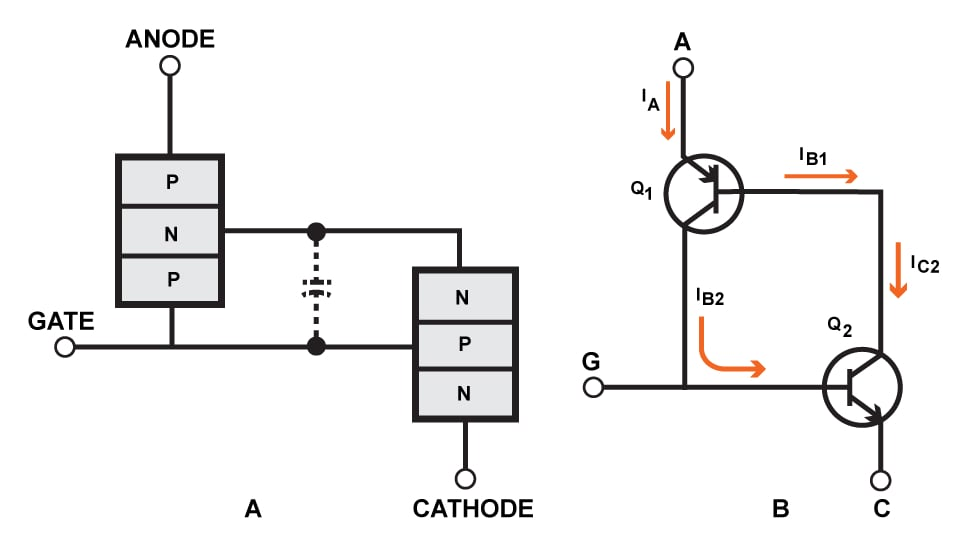##### Figure 8. The PNPN structure acts like two BJT transistors. Image adapted from Solid-state Relay Handbook with Applications by Anthony Bishop.

When the gate voltage of Figure 8(B) increases, $$I_{C2}$$ increases which leads to an increase in $$I_{B2}$$. This in turn further increases the gate voltage of Q2. As a result, there is a positive feedback mechanism which tries to increase the anode-to-cathode current of the SCR. While we can turn the SCR on by applying a signal to its gate, we cannot turn it off by controlling the gate. The SCR can only be turned off by reducing the current flowing into the device below a certain level so that the loop gain of the positive feedback goes below unity and the device stops conducting. Due to this feedback mechanism, we cannot use SCRs to switch a DC load on and off.

Since an SCR conducts for only half the cycle, to switch an AC power supply it is necessary to put a single SCR in a bridge configuration or use two SCRs in inverse parallel configuration, as shown in Figure 9.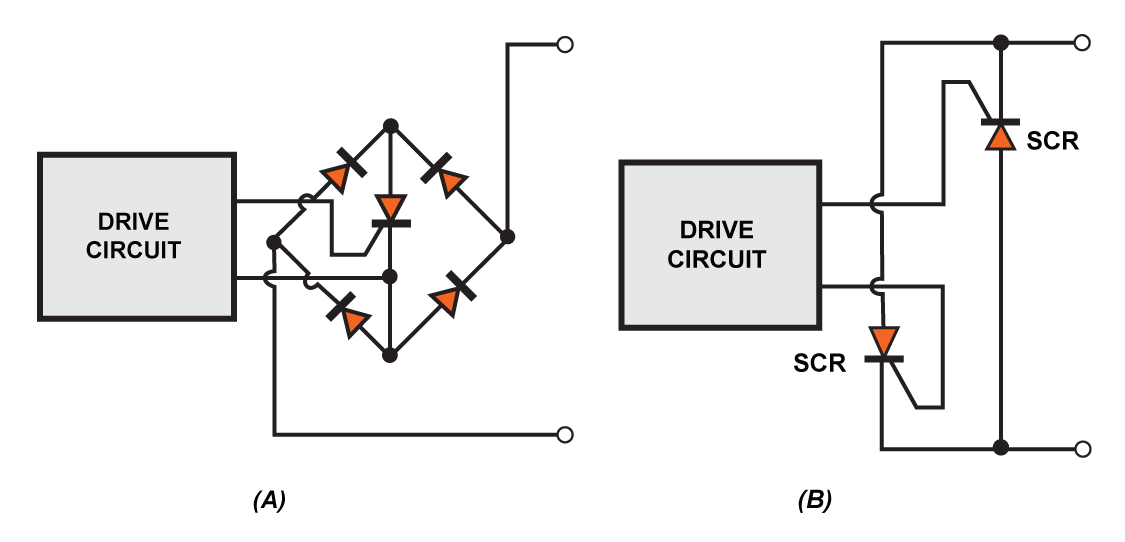##### Figure 9. An SCR-based SSR can be obtained by putting one SCR in a bridge (A) or two SCRs in inverse parallel (B). Image adapted from Solid-state Relay Handbook with Applications by Anthony Bishop.

In Figure 9(A), when the SCR is on, the bridge structure provides a path for the current to flow no matter what the polarity of the power supply is. One of the main drawbacks to this circuit is the voltage drop from two additional diodes which must be added to that of the SCR.  The structure shown in Figure 9(B) does not introduce additional power loss but needs two controlling signals to operate the SCRs. One way to produce these two controlling signals is to use a variation of the circuit shown in Figure 9(A) as the driving stage of Figure 9(B). The overall structure will be very similar to the one shown in Figure 11. Note that the structure in Figure 9(A) is suitable for use as a driver for another switching device because in a prior stage the current can be much lower and the power consumption is manageable.

By integrating the two SCRs into an inverse parallel structure, manufacturers achieve a true AC switch called a TRIAC. A TRIAC has three terminals: gate, main terminal 2, and main terminal 1. Interestingly, a TRIAC has only one control-signal input, and when the device is activated via this gate terminal, it conducts in either direction. The overall switching characteristic of a TRIAC is similar to that of two SCRs in inverse parallel configuration.

As shown in Figure 10, a TRIAC can be used as the switching device of an SSR. However, it is possible to use the TRIAC as a driver for another switching device, as shown in Figure 11. This scheme allows a low-power SSR to control high-current loads by means of external, high-power SCRs. Figure 11 shows how we can use a TRIAC along with three resistors to produce the two controlling signals of Figure 9(B). The reader can verify that, regardless of the polarity of the output AC voltage, the gate of the SCRs will have a positive voltage due to the current which goes through the TRIAC. Now, based on the polarity of the AC voltage one of the two SCRs will conduct the current.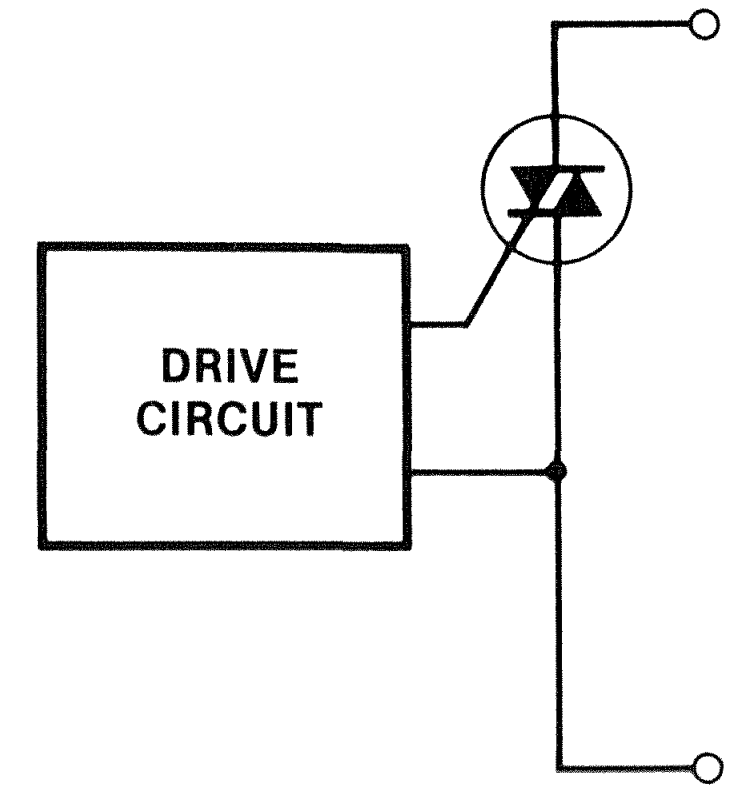##### Figure 10. A TRIAC as the output device of an SSR. Image courtesy of Solid-state Relay Handbook with Applications by Anthony Bishop.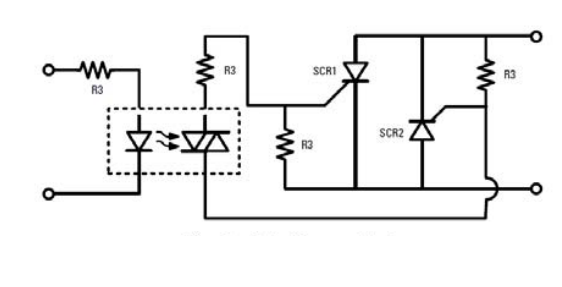##### Figure 11. Use of a TRIAC as the driver for two SCRs in inverse parallel configuration. Image courtesy of Crydom.• R
ronsoy2 June 28, 2017

One problem is many SSR do not specify which type of switching element is used. SCR and TRIAC types cannot easily switch AC inductive loads without carefully designed external snubbing circuitry. The FET types can. For a given power rating the FET types are noticeably more expensive than the TRIAC type, but still may be lower cost than a mechanical contactor of the same rating.

Like.
• S
snydert October 05, 2017

Would like to see more on the topics of Zero Crossing Switching, and just how quickly, (frequency) an SSR can be cycled.

Like.
• L
lonnie77 November 07, 2017

Good information. Now, how do you troubleshoot a SSR?

Like.